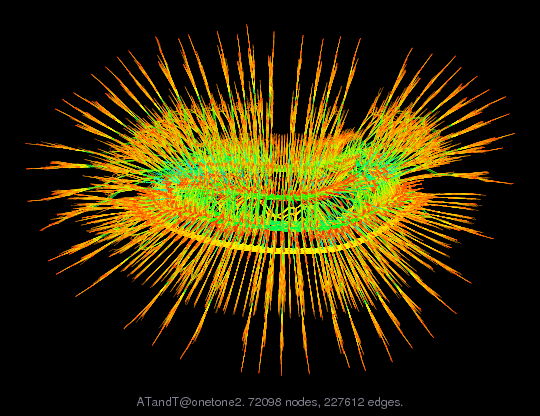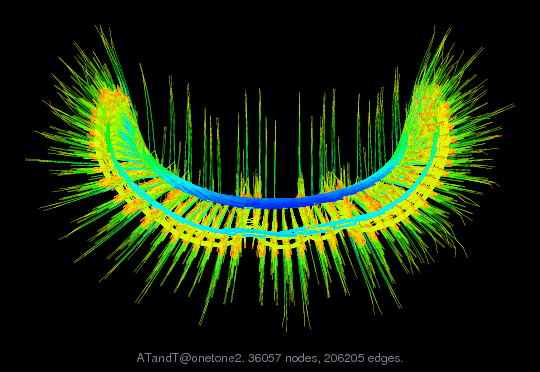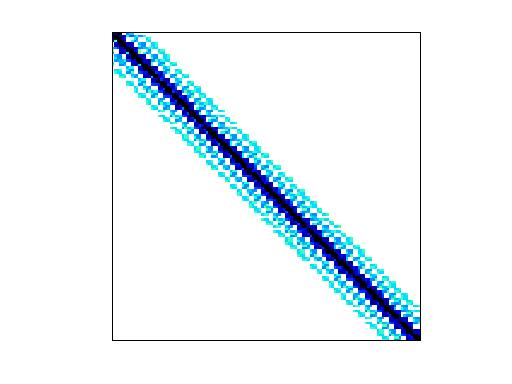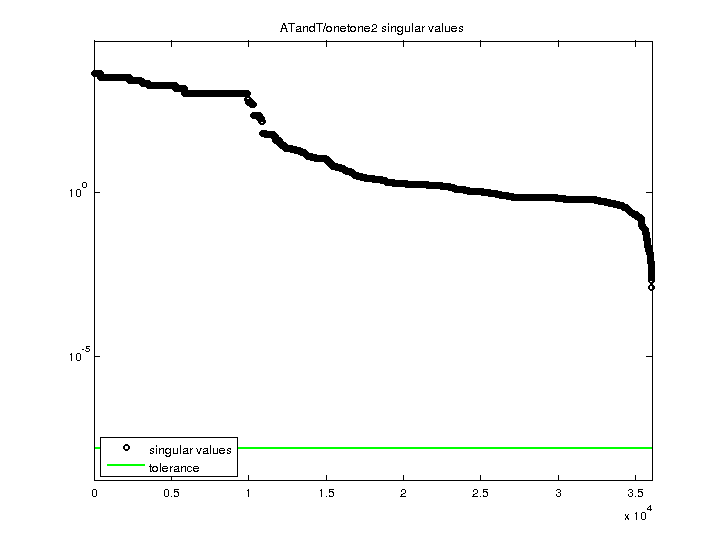Matrix: ATandT/onetone2

Description: AT&T,harmonic balance method, one-tone. More typical for direct factor.(bipartite graph drawing) (graph drawing of A+A')• Matrix group: ATandT
• download as a MATLAB mat-file, file size: 1 MB. Use UFget(284) or UFget('ATandT/onetone2') in MATLAB.

 Matrix properties number of rows 36,057 number of columns 36,057 nonzeros 222,596 structural full rank? yes structural rank 36,057 # of blocks from dmperm 3,843 # strongly connected comp. 203 explicit zero entries 5,032 nonzero pattern symmetry 11% numeric value symmetry 7% type real structure unsymmetric Cholesky candidate? no positive definite? no

 author R. Melville, D. Long editor T. Davis date 2001 kind frequency-domain circuit simulation problem 2D/3D problem? no

 Ordering statistics: result nnz(chol(P*(A+A'+s*I)*P')) with AMD 4,018,036 Cholesky flop count 1.0e+09 nnz(L+U), no partial pivoting, with AMD 8,000,015 nnz(V) for QR, upper bound nnz(L) for LU, with COLAMD 488,470 nnz(R) for QR, upper bound nnz(U) for LU, with COLAMD 1,065,910

Note that all matrix statistics (except nonzero pattern symmetry) exclude the 5032 explicit zero entries.

 SVD-based statistics: norm(A) 3924.01 min(svd(A)) 0.00124 cond(A) 3.16452e+06 rank(A) 36,057 sprank(A)-rank(A) 0 null space dimension 0 full numerical rank? yes

 singular values (MAT file): click here SVD method used: s = svd (full (A)) status: ok